# Whitney-Graustein theorem

(diff) ← Older revision | Latest revision (diff) | Newer revision → (diff)

The winding number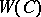of a continuous closed curvein the plane, missing the origin, counts the algebraic number of timesgoes around the origin in the counterclockwise direction. Ifis a regular closed curve in the plane, i.e., ifis continuously differentiable and has non-vanishing derivative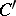, then the degree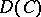ofis defined to be the winding number of. Thus,counts the algebraic number of turns ofin the counterclockwise direction.

The Whitney–Graustein theorem states that regular homotopy classes of regular curves in the plane are completely classified by the degree, i.e., two regular curves in the plane are regularly homotopic if and only if their degrees are equal (see [a2]; cf. also Homotopy). Furthermore, in [a2] H. Whitney also shows that the degree and the self-intersection number have different parity.

The above definition of degree can be extended to a larger class of curves. If a continuous closed planar curveis locally one-to-one, then its secant vectors corresponding to pairs of points onthat are close enough, are non-zero. Therefore, the winding number of the path defined by secant vectors can be used, as before, to define the degree of. More precisely,is the winding number of the curve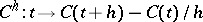, forsmall enough, or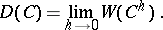Replacing regular homotopy by gentle homotopy, i.e., by a homotopy which is locally one-to-one, one gets the following analogue of the Whitney–Graustein Theorem: The gentle homotopy classes of locally one-to-one curves in the plane are completely classified by the degree, i.e., two locally one-to-one curves in the plane are gently homotopic if and only if their degrees are equal (see [a1]). In this case the degree and the self-intersection number have different parity as well.

The Whitney–Graustein theorem implies that the tangent vectors of any regular closed curve in the plane point in each direction at least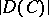times. It is interesting to note that this is true even for differentiable locally one-to-one curves which are not necessarily continuously differentiable (see [a1]).

How to Cite This Entry:
Whitney-Graustein theorem. Encyclopedia of Mathematics. URL: http://encyclopediaofmath.org/index.php?title=Whitney-Graustein_theorem&oldid=16207
This article was adapted from an original article by Marko Kranjc (originator), which appeared in Encyclopedia of Mathematics - ISBN 1402006098. See original article